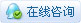5273浏览

# [项目] 制作Arduino 超声波雷达

 ## 制作Arduino超声波雷达 接下来就要开始制作Arduino 超声波雷达了。你要准备好如下材料和工具： 超声波传感器×1 舵机×1 杜邦线导线若干 软件用的是arduino ide和processingarduino IDE代码： [mw_shl_code=applescript,true]#include const int trigPin = 10; const int echoPin = 11; long duration; int distance; Servo myServo; void setup() {     pinMode(trigPin, OUTPUT);     pinMode(echoPin, INPUT);     Serial.begin(9600);     myServo.attach(12); } void loop() {     for(int i=15;i<=165;i++){         myServo.write(i);         delay(30);         distance = calculateDistance();         Serial.print(i);         Serial.print(",");         Serial.print(distance);         Serial.print(".");     }         for(int i=165;i>15;i--){         myServo.write(i);         delay(30);         distance = calculateDistance();         Serial.print(i);         Serial.print(",");         Serial.print(distance);         Serial.print(".");     } } int calculateDistance(){     digitalWrite(trigPin, LOW);     delayMicroseconds(2);        digitalWrite(trigPin, HIGH);     delayMicroseconds(10);     digitalWrite(trigPin, LOW);     duration = pulseIn(echoPin, HIGH);     distance= duration*0.034/2;     return distance; } processing代码： import processing.serial.*; // imports library for serial communication import java.awt.event.KeyEvent; // imports library for reading the data from the serial port import java.io.IOException; Serial myPort; // defines Object Serial // defubes variables String angle=""; String distance=""; String data=""; String noObject; float pixsDistance; int iAngle, iDistance; int index1=0; int index2=0; PFont orcFont; void setup() {    size (1000, 720); // ***CHANGE THIS TO YOUR SCREEN RESOLUTION*** smooth(); myPort = new Serial(this,"COM6", 9600); // starts the serial communication myPort.bufferUntil('.'); // reads the data from the serial port up to the character '.'. So actually it reads this: angle,distance. orcFont = loadFont("AgencyFB-Bold-48.vlw"); } void draw() {      fill(98,245,31);   textFont(orcFont);   // simulating motion blur and slow fade of the moving line   noStroke();   fill(0,4);   rect(0, 0, width, height-height*0.065);      fill(98,245,31); // green color   // calls the functions for drawing the radar   drawRadar();   drawLine();   drawObject();   drawText(); } void serialEvent (Serial myPort) { // starts reading data from the Serial Port   // reads the data from the Serial Port up to the character '.' and puts it into the String variable "data".   data = myPort.readStringUntil('.');   data = data.substring(0,data.length()-1);      index1 = data.indexOf(","); // find the character ',' and puts it into the variable "index1"   angle= data.substring(0, index1); // read the data from position "0" to position of the variable index1 or thats the value of the angle the Arduino Board sent into the Serial Port   distance= data.substring(index1+1, data.length()); // read the data from position "index1" to the end of the data pr thats the value of the distance      // converts the String variables into Integer   iAngle = int(angle);   iDistance = int(distance); } void drawRadar() {   pushMatrix();   translate(width/2,height-height*0.074); // moves the starting coordinats to new location   noFill();   strokeWeight(2);   stroke(98,245,31);   // draws the arc lines   arc(0,0,(width-width*0.0625),(width-width*0.0625),PI,TWO_PI);   arc(0,0,(width-width*0.27),(width-width*0.27),PI,TWO_PI);   arc(0,0,(width-width*0.479),(width-width*0.479),PI,TWO_PI);   arc(0,0,(width-width*0.687),(width-width*0.687),PI,TWO_PI);   // draws the angle lines   line(-width/2,0,width/2,0);   line(0,0,(-width/2)*cos(radians(30)),(-width/2)*sin(radians(30)));   line(0,0,(-width/2)*cos(radians(60)),(-width/2)*sin(radians(60)));   line(0,0,(-width/2)*cos(radians(90)),(-width/2)*sin(radians(90)));   line(0,0,(-width/2)*cos(radians(120)),(-width/2)*sin(radians(120)));   line(0,0,(-width/2)*cos(radians(150)),(-width/2)*sin(radians(150)));   line((-width/2)*cos(radians(30)),0,width/2,0);   popMatrix(); } void drawObject() {   pushMatrix();   translate(width/2,height-height*0.074); // moves the starting coordinats to new location   strokeWeight(9);   stroke(255,10,10); // red color   pixsDistance = iDistance*((height-height*0.1666)*0.025); // covers the distance from the sensor from cm to pixels   // limiting the range to 40 cms   if(iDistance<40){     // draws the object according to the angle and the distance   line(pixsDistance*cos(radians(iAngle)),-pixsDistance*sin(radians(iAngle)),(width-width*0.505)*cos(radians(iAngle)),-(width-width*0.505)*sin(radians(iAngle)));   }   popMatrix(); } void drawLine() {   pushMatrix();   strokeWeight(9);   stroke(30,250,60);   translate(width/2,height-height*0.074); // moves the starting coordinats to new location   line(0,0,(height-height*0.12)*cos(radians(iAngle)),-(height-height*0.12)*sin(radians(iAngle))); // draws the line according to the angle   popMatrix(); } void drawText() { // draws the texts on the screen      pushMatrix();   if(iDistance>40) {   noObject = "Out of Range";   }   else {   noObject = "In Range";   }   fill(0,0,0);   noStroke();   rect(0, height-height*0.0648, width, height);   fill(98,245,31);   textSize(25);      text("10cm",width-width*0.3854,height-height*0.0833);   text("20cm",width-width*0.281,height-height*0.0833);   text("30cm",width-width*0.177,height-height*0.0833);   text("40cm",width-width*0.0729,height-height*0.0833);   textSize(40);   text("Object: " + noObject, width-width*0.875, height-height*0.0277);   text("Angle: " + iAngle +" °", width-width*0.48, height-height*0.0277);   text("Distance: ", width-width*0.26, height-height*0.0277);   if(iDistance<40) {   text("        " + iDistance +" cm", width-width*0.225, height-height*0.0277);   }   textSize(25);   fill(98,245,60);   translate((width-width*0.4994)+width/2*cos(radians(30)),(height-height*0.0907)-width/2*sin(radians(30)));   rotate(-radians(-60));   text("30°",0,0);   resetMatrix();   translate((width-width*0.503)+width/2*cos(radians(60)),(height-height*0.0888)-width/2*sin(radians(60)));   rotate(-radians(-30));   text("60°",0,0);   resetMatrix();   translate((width-width*0.507)+width/2*cos(radians(90)),(height-height*0.0833)-width/2*sin(radians(90)));   rotate(radians(0));   text("90°",0,0);   resetMatrix();   translate(width-width*0.513+width/2*cos(radians(120)),(height-height*0.07129)-width/2*sin(radians(120)));   rotate(radians(-30));   text("120°",0,0);   resetMatrix();   translate((width-width*0.5104)+width/2*cos(radians(150)),(height-height*0.0574)-width/2*sin(radians(150)));   rotate(radians(-60));   text("150°",0,0);   popMatrix(); }[/mw_shl_code] 代码文件下载地址： https://pan.baidu.com/s/1gLW7nad-az-g0Pod7k2gRQ### nene学徒

 本帖最后由 nene 于 2019-10-16 08:42 编辑 这个用processing比较多，Arduino部分倒是很简单的控制 我明白了，这个是相当于用processing作为上位机处理和显示数据。 可惜processing不熟悉，是不是雷达传感器就是这个意思？

 processing也是个选择

 求电路图 原理图 谢谢

 您需要登录后才可以回帖 登录 | 立即注册 本版积分规则 回帖并转播 回帖后跳转到最后一页

[[wsData.name]]

#### 硬件清单

• [[d.name]]#### 楼主的其它帖子